Courses

# Test: Heat Dissipation From Extended Surface - 2

## 10 Questions MCQ Test Topicwise Question Bank for Mechanical Engineering | Test: Heat Dissipation From Extended Surface - 2

Description
This mock test of Test: Heat Dissipation From Extended Surface - 2 for Mechanical Engineering helps you for every Mechanical Engineering entrance exam. This contains 10 Multiple Choice Questions for Mechanical Engineering Test: Heat Dissipation From Extended Surface - 2 (mcq) to study with solutions a complete question bank. The solved questions answers in this Test: Heat Dissipation From Extended Surface - 2 quiz give you a good mix of easy questions and tough questions. Mechanical Engineering students definitely take this Test: Heat Dissipation From Extended Surface - 2 exercise for a better result in the exam. You can find other Test: Heat Dissipation From Extended Surface - 2 extra questions, long questions & short questions for Mechanical Engineering on EduRev as well by searching above.
QUESTION: 1

### Consider the following statements regarding fin of minimum weight 1. The weight saved by using the triangular fin is 44%. 2. The difference in weight between a fin in shape of circular arc and the fin of triangular shape is very large. 3. The triangular shape fin is regarded as best shape as it is much easier to manufacture. 4. Weight of fin is of paramount importance for the design of cooling device used in aircraft. Which of the above are correct?

Solution:

The difference in weight between a fin in shape of circular arc and the fin of triangular shape is very small.

QUESTION: 2

### Fin efficiency is defined as the ratio of the heat transferred across the fin surface to the theoretical heat transfer across an equal area held at

Solution: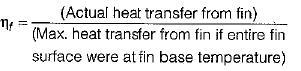QUESTION: 3

### Usually fins are provided to increase the rate of heat transfer but fin also acts as insulation which one of the following non-dimensional number decides this factor

Solution:
QUESTION: 4

The effectiveness of a fin will be maximum in an environment with

Solution:

Effectiveness of the fin is given by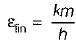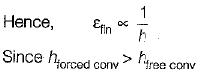Hence, Effectiveness of the fin will be more in free convection than forced convection.

QUESTION: 5

Fins are used to increase the heat transfer rate from surface by

Solution:

Since area is crucial in any of the mode of heat transfer hence by increasing effective surface area heat transfer rate can be enhanced.

QUESTION: 6

Efficiency of a fin with insulated tip is

Solution:

Efficiency of fin with insulated tip =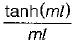QUESTION: 7

The insulated tip temperature of a rectangular longitudinal fin having an excess (over ambient root temperature of θ0) is

Solution:

Temperature distribution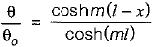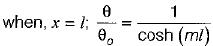QUESTION: 8

A thermocouple in a thermowell measures the temperature of hot gas flowing through the pipe for the most accurate measurement of temperature, the thermowell should be made of

Solution:
QUESTION: 9

Consider the following statement pertaining to large heat transfer rate using fins
1. Fins should be used on the side where heat transfer coefficient is small
2. Long and thick fins should be used
3. Short and thin fins should be used
4. Thermal conductivity of fin material should be large

Which of the above statements are correct ?

Solution:
QUESTION: 10

Addition of fin to the surface increases the heat transfer. It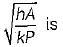Solution: